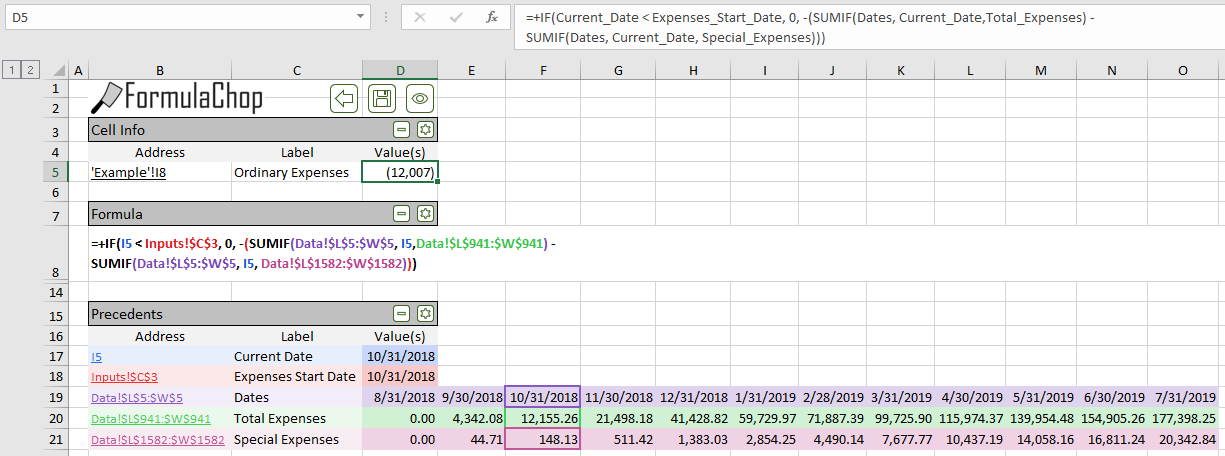# Introduction¶

## What is FormulaChop?¶

FormulaChop is an Excel VBA Add-In to help with understanding formulas

## Why was FormulaChop Created?¶

FormulaChop was created to fill a specific need: quickly, easily understand sprawling, complex Excel spreadsheets.

We often receive Excel spreadsheets which are very large and complex. We would look at a value in one of these spreadsheets and then ask, “Where does this number come from?” Often, the formula for this value would be long, complicated, and referring to far-away ranges. Excel’s built-in formula auditing tools helped, but do not handle certain common functions in a useful way. We would need to go find the precedent ranges of formulas by hand. All of this made auditing and understanding these spreadsheets tedious, difficult and time-consuming.

FormulaChop has completely changed our process for auditing spreadsheets. Any spreadsheet, complex or simple, is easy and quick to understand.

## What Does FormulaChop Do?¶

FormulaChop helps with understanding spreadsheets in two key ways:

• By generating a detailed report for a given cell
• By making it very easy to navigate between precedents and dependents, across the entire workbook

## FormulaChop Output¶A sample FormulaChop Tab

### Cell Info¶

The Cell Info section shows information about the Chopped Cell, which is the cell on which FormulaChop was run

### Formula¶

The Formula section shows the formula FormulaChop was run on, with ranges color-coded

### Precedents¶

The Precedents section shows the values that the formula refers to, along with their label